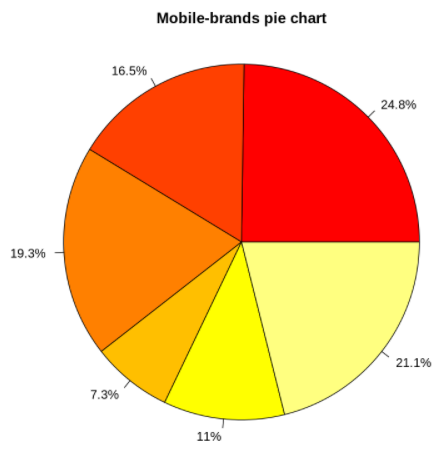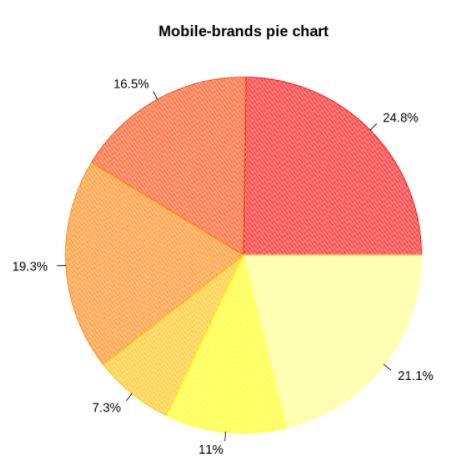# Tutorial of Pie Chart in Base R Programming Language### Example 5: Add Color Pallete to Pie Chart

Instead of applying colors to each slice, the color palette can be added to the complete pie chart as shown in this example.
In :
Out:### Example 6: Hatch Fill Pie Chart

The hatch fill pattern can be applied to Pie Chart by using density and angle parameters.
In:
```perc_value<- round(100 * units / sum(units), 1)

# Plot the chart
pie(units, labels = paste0(perc_value, "%"), radius=1,
main = "Mobile-brands pie chart",
col=heat.colors(6), density = 60, angle = 75)```
Out: